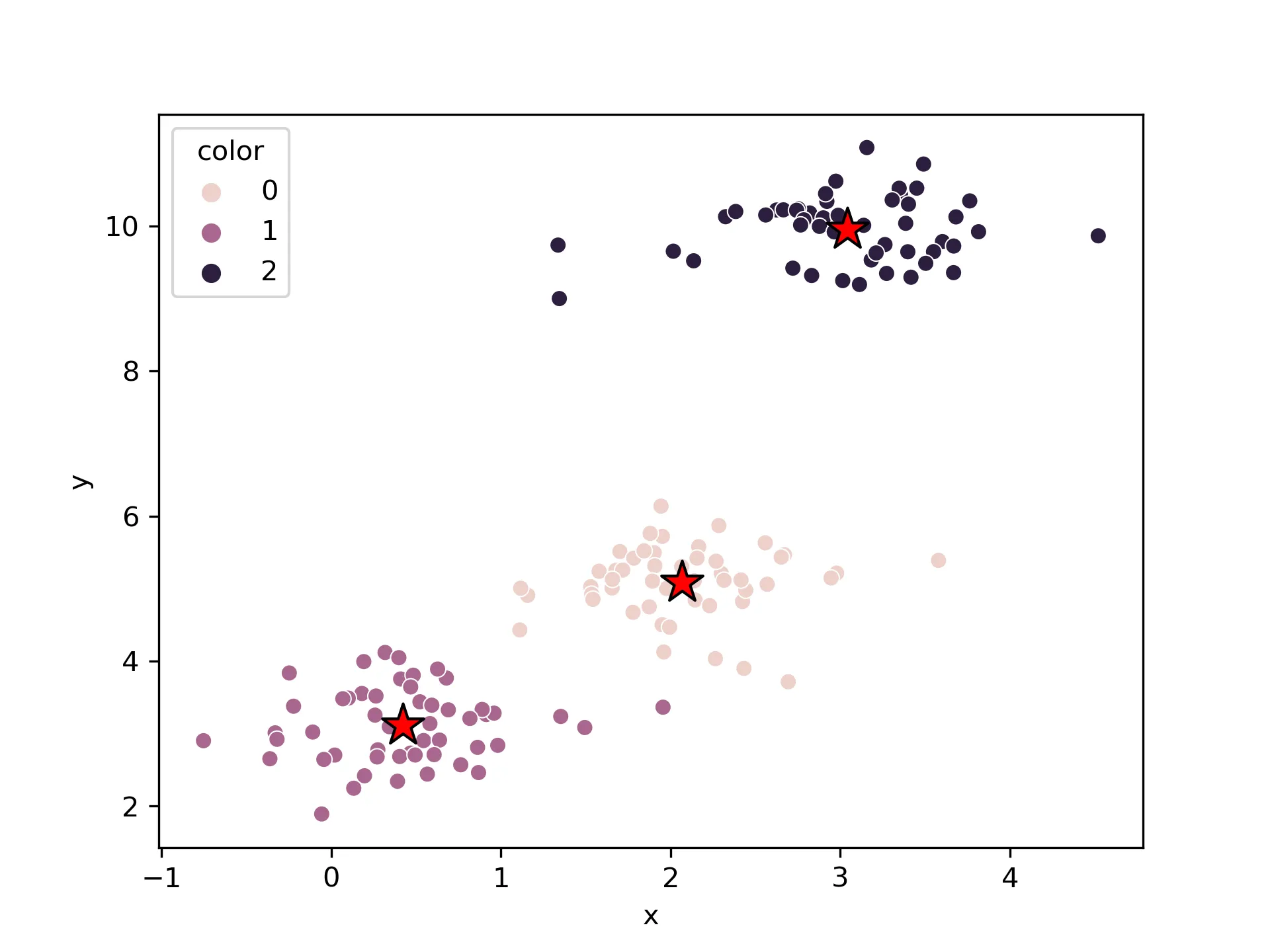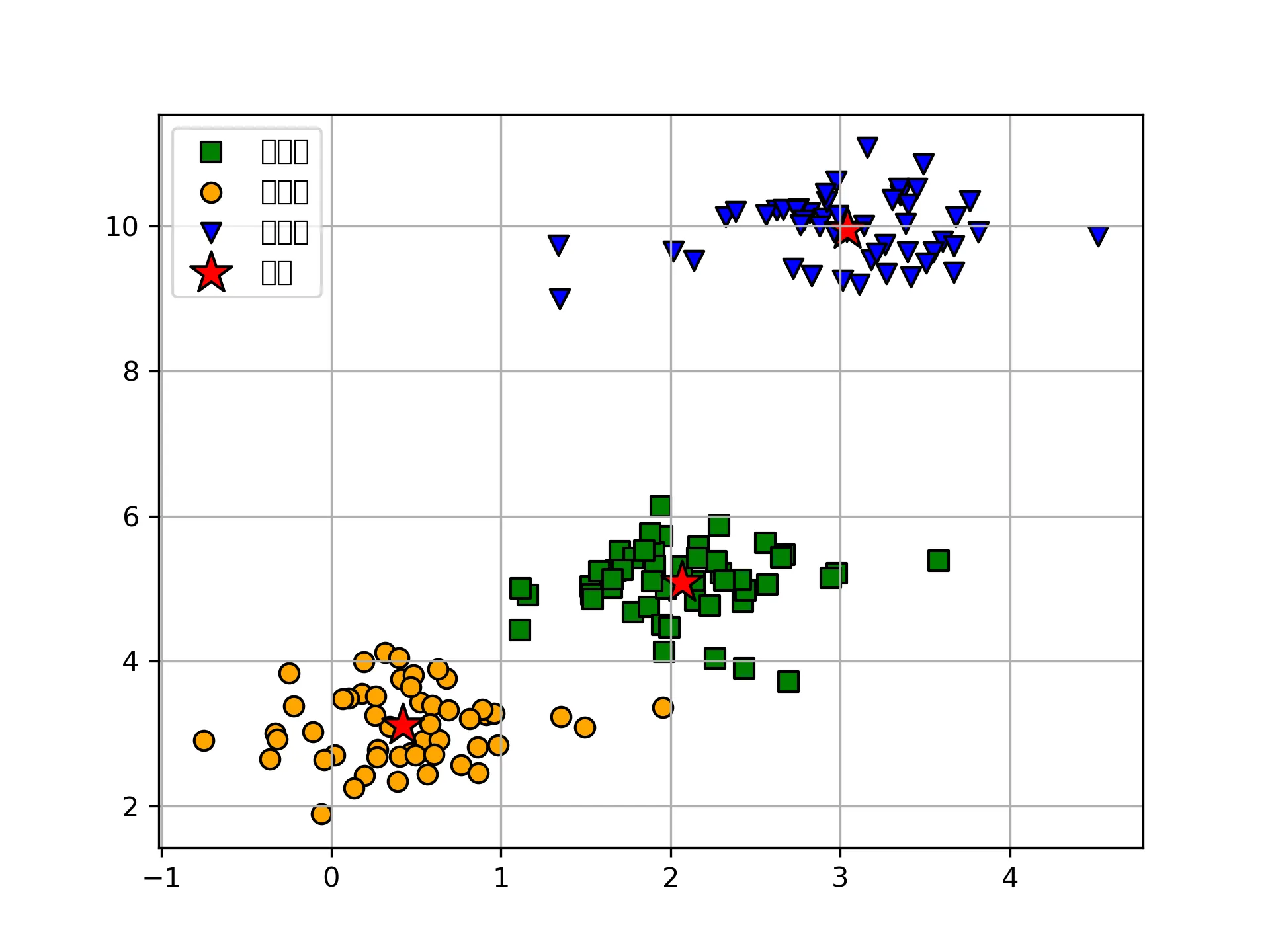• k-means聚类的基本概念
• k-means 算法背后的数学原理
• k-means的优缺点
• 使用scikit-learn 包实现
• 可视化分类
• 选择最优的k

## k-means聚类的基本概念

k-means是一种高效的无监督的聚类方法，最初用于信号处理，旨在将n个观测值划分为k个簇，其中每个观测值都属于具有最近均值的簇（簇中心或簇质心），作为群。很容易混淆的另一种聚类方法是监督学习算法k-nearest neighbors (KNN)，需要注意。

## k-means的优缺点

1. 原理简单，实现快
2. 聚类效果好
3. 算法可解释度高
4. 只需要调整聚类个数k

1. K值的选取不好把握
2. 对于不是凸的数据集比较难收敛
3. 采用迭代方法，得到的结果只是局部最优
4. 对噪音和异常敏感

## 使用scikit-learn 包实现

import matplotlib.pyplot as plt
import pandas as pd
import seaborn as sns
from sklearn.datasets import make_blobs
from sklearn.cluster import KMeans

# from 春江暮客

x,y = make_blobs(n_samples=150, n_features=2,centers=3, cluster_std=0.5,shuffle=True, random_state=1024)
km = KMeans( n_clusters=3, init='random',n_init=10, max_iter=300, tol=1e-04, random_state=1024)
y_km = km.fit_predict(x)



## 可视化分类

# seaborn

df = pd.DataFrame(x,columns=["x","y"])
df["color"] = y_km
sns.scatterplot(data=df,x="x",y="y",hue="color", markers="color")
# 绘制聚类中心
plt.scatter(
km.cluster_centers_[:, 0], km.cluster_centers_[:, 1],
s=250, marker='*',
c='red', edgecolor='black',
label='center'
)# matplotlib
plt.scatter(
x[y_km == 0, 0], x[y_km == 0, 1],
s=50, c='green',
marker='s', edgecolor='black',
label='cluster1'
)

plt.scatter(
x[y_km == 1, 0], x[y_km == 1, 1],
s=50, c='orange',
marker='o', edgecolor='black',
label='cluster2'
)

plt.scatter(
x[y_km == 2, 0], x[y_km == 2, 1],
s=50, c='blue',
marker='v', edgecolor='black',
label='cluster3'
)

# 绘制聚类中心
plt.scatter(
km.cluster_centers_[:, 0], km.cluster_centers_[:, 1],
s=250, marker='*',
c='red', edgecolor='black',
label='center'
)
plt.legend(scatterpoints=1)
plt.grid()
plt.show()## 选择最优的k

distortions = []
for i in range(1, 11):
km = KMeans(
n_clusters=i, init='random',
n_init=10, max_iter=300,
tol=1e-04, random_state=1024
)
km.fit(x)
distortions.append(km.inertia_)

# 绘图
plt.plot(range(1, 11), distortions, marker='o')
plt.xlabel('Number of clusters')
plt.ylabel('Distortion')
plt.show()